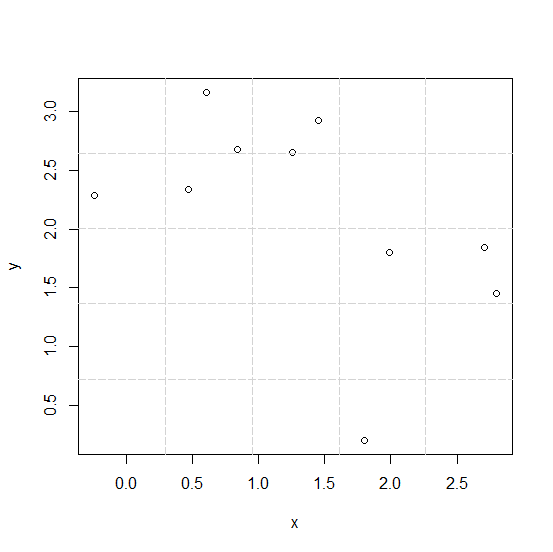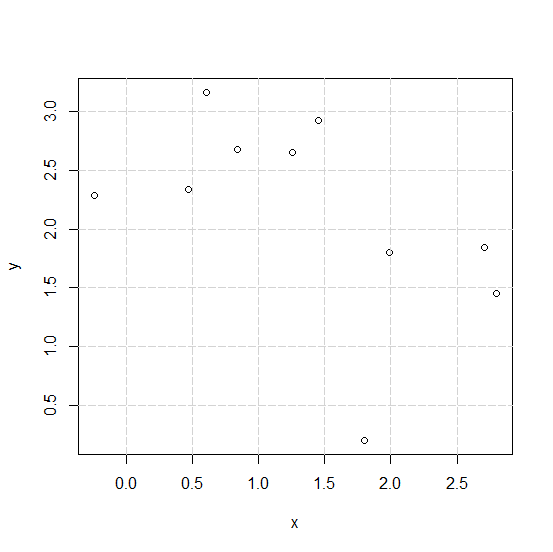# How to create gridlines that matches with Y-axis values in the plot created by using plot function in R?

When we create a plot in R and draw gridlines then the gridlines are drawn on the basis of the values provided inside the grid function, therefore, it may or may not match with the Y-axis labels. But it can be done, we just need to set the values inside the grid function to NULL.

## Example

Consider the below plot −

Live Demo

x<-rnorm(10,1)
y<-rnorm(10,2)
plot(x,y)
grid(5,5,lty=5)

## OutputCreating the plot with gridlines that matches with Y-axis labels −

## Example

plot(x,y)
grid(NULL,NULL,lty=5)

## Output